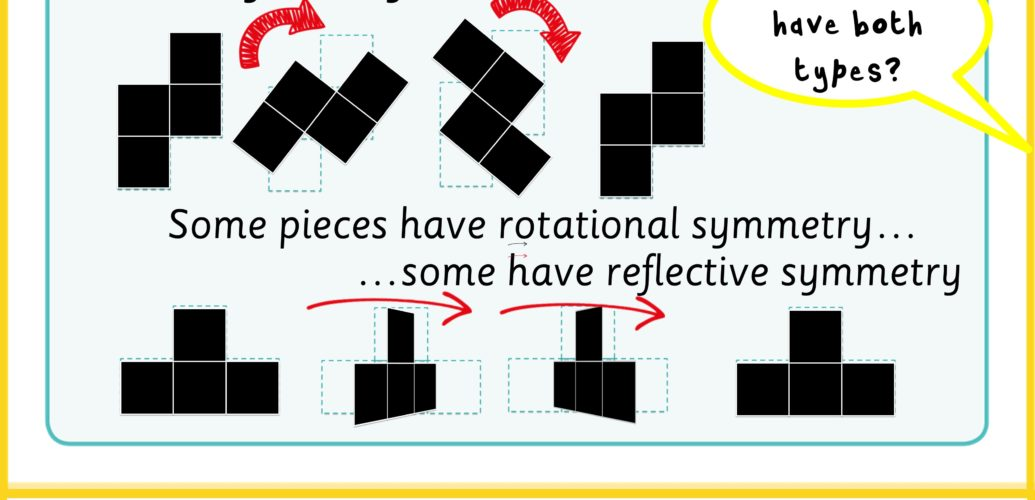# Polyominoes and Symmetry

One of the difficulties in learning maths, and especially geometry is that we learn about mathematical objects in non mathematical ways. So we might learn algebra by learning to move numbers around the equation. This might get you the answer to a question but it will stop you developing your understanding.

Shape is another area where this can be a difficulty. We learn about shapes based on the way they look or more correctly, the way they are presented. In maths, it is the properties of the shape that count rather than the way they look. So it can be that a student does not see a shape as a triangle simply because it is not pointing up.

Symmetry is a key property of a shape and getting used to seeing the different symmetries will benefit you/your children.

#### Rotational Symmetry

Rotate a shape in its plane i.e. on the sheet, and if it matches the original shape before you get all the way round, it’s rotationally symmetrical.

#### Reflective Symmetry

If you can flip a shape over on to the other side and it matches the original, then it has reflective symmetry. Another way to think about this is that if you look at the object in the mirror, the reflection is exactly the same shape.

#### Carroll Diagrams

These diagrams were invented by Lewis Carroll (real name Charles Lutwidge Dodgson) who wrote Alice in Wonderland. Well they are not much of an invention, but they are remarkably useful as a way of thinking about categories.

We start by taking a category such as rotational symmetry. A shape is either rotationally symmetrical or not so we can always sort a shame in one or another category. Then if we have another category like reflective symmetry, we can sort that on a different axis. This gives us a grid with 4 quadrants that mean we have 4 categories for our shapes. Each shape may only have both types of symmetry, just one of the types or no symmetry at all.

This week’s challenge is to find all the pentominoes that use 4 squares or less and sort them using the Carroll diagram. There are 9 of these shapes in all.